Courses

# Chapter 4 - Work, Power and Energy Notes | EduRev

## JEE : Chapter 4 - Work, Power and Energy Notes | EduRev

The document Chapter 4 - Work, Power and Energy Notes | EduRev is a part of JEE category.
All you need of JEE at this link: JEE

Work

• Whenever a force acting on a body is able to actually move it through some distance in the direction of force, the work is said to be done by the force.
• When a constant force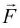acts on a particle while it moves through a displacement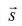, the force is said to do work W on the particle given by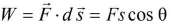where θ is the smaller angle between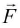and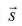.
• If the force and displacement are in the same direction, θ = 0.
∴ W = Fs.
• If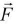and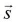are perpendicular to each other, then,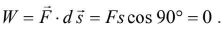This means that if the displacement of the body is perpendicular to the force no work is done.
• If the force and displacement are variable quantities, then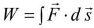= area under the force displacement graph, added with proper sign
• Work is a scalar quantity.
• In SI system the absolute unit of work is joule, while in CGS system, the unit of work is erg.
• Dimensions of work are
W= Fs = [MLT-2] [L] = [ML2T-2].
Workdone by a constant force in moving from one point to other depends only on the initial and final positions and not on the actual path followed between two points.
• If the workdone by a force during a round trip of a system is zero, the force is said to be conservative, otherwise it is called non-conservative. Force of friction is a non-conservative force.
• Workdone in moving an object against gravity depends only on the initial and final positions of the object but not upon the path taken. This shows that gravitational force is conservative force.
• All the central forces are conservative forces.
• The workdone by the force of gravity during the motion of a particle through a height h is W= mgh, where m is the mass of the particle.
• Workdone in compressing/stretching a spring,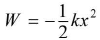where k is spring constant and x is the displacement from normal position of rest.
• When one end of a spring is attached to a fixed vertical support and a block attached to the free end moves on a horizontal table from x = to x1 = x2, then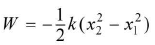• Workdone by the couple for an angular displacement of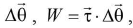where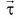is the torque of couple.

Power

• Power is the time rate of doing work. Thus, Power =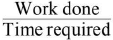• If a constant force F acts on a body for time t and if due to this the body moves a distance 5 in the direction of the force, with uniform velocity v, then power P developed is given by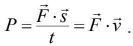Thus P = F v cos θ
Here θ is the smaller angle between force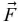and velocity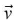of the body.
• Power is a scalar quantity. SI unit of power is watt where 1 watt = 1 joule/sec.
• The practical unit of power is horse power (hp), where 1 hp = 746 watt.
• Dimensions of power are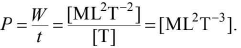ENERGY

• Energy is the capacity of a body for doing work, i.e. it is the total amount of work stored up in the body.
• Because the energy of a body represents the total amount of workdone, hence
(a) it is a scalar quantity just like work.
(b) its dimensions are the same as those of work,
i.e. [ML2T-2].
(c) it is measured in the same units as the work, i.e. joule and erg.
• Energy can exist in different forms such as mechanical energy, heat energy, sound energy, electrical energy, light energy, etc.
• The mechanical energy is of two types - kinetic energy and potential energy.
• Kinetic energy of a body is the energy acquired by the body by virtue of its motion.
• Kinetic energy is measured by the workdone by the moving body against external impressed force, before coming to rest. If a body of mass m moves with velocity v, its kinetic energy is given by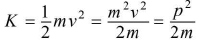where p = mv = momentum of the body
• Potential energy of a body is the energy possessed by the body by virtue of its position or configuration. The potential energy is due to the interaction between bodies.
• If a body of mass m be raised through a vertical height h against gravity, then its gravitational potential energy is given as
potential energy = mgh.
• Potential energy can be defined only for conservative forces. It does not exist for non-conservative forces. A moving body may or may not have potential energy. Further, potential energy does depends upon the frame of reference.
• Workdone by a force acting on a body is equal to change in kinetic energy of the body This is called as work energy of the body. This is called as work-energy theorem. Mathematically, work-energy theorem can be written as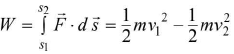• For conservative forces the sum of kinetic and potential energies at any point remains constant throughout the motion. It does not depend upon time. This is known as law of conservation of mechanical energy.

Collisions

• Collision between two particles is defined as the mutual interaction of the particle for a small interval of time due to which both the energy and momentum of the interacting particles change.
• In physics a collision will take place if either of the two bodies come in physical contact with each other or even when path of one body is affected by the force exerted due to the other.
• Collisions are of two types:
(a) The collisions, in which both the momentum and kinetic energy of the system remain conserved, are called elastic collisions.
(b) The collisions in which only the momentum of the system is conserved but kinetic energy is not conserved are called inelastic collisions.
• Linear momentum is conserved in both elastic as well as inelastic collision.
• If the initial and final velocities of colliding masses lie along the same line, then it is known as head-on collision or one dimensional collision.
• If the velocities of the colliding masses are not collinear, then it is known as oblique collision or two dimensional collision.
• In one dimensional elastic collision, the relative velocity of approach (u1 - u2) before collision is equal to the relative velocity of separation (v2 - v1) after collision.
• Velocity of the two bodies after collision;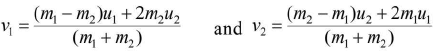• If two bodies of equal masses undergo elastic collision in one dimension, then after the collision, the bodies will exchange their velocities.
• Maximum transfer of energy occurs when
(a) target body is at rest initially
(b) masses of two bodies are equal.
• The loss of kinetic energy in perfectly inelastic collision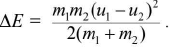• For a perfectly elastic collision,
velocity of separation = velocity of approach
For a perfectly inelastic collision,
velocity of separation = 0.
• In general, the bodies are neither perfectly elastic nor perfectly inelastic. In that case we write:
velocity of separation = e (velocity of approach)
where 0 < e < 1.
• The constant e depends upon the material of the colliding bodies. This constant is known as coefficient of restitution. If e = 1, the collision is perfectly elastic and if e = 0, the collision is perfectly inelastic.
• General expression for velocities after direct impact are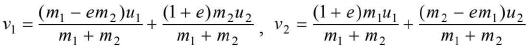• The loss in kinetic energy of two bodies after an inelastic collision,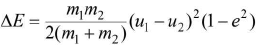• Suppose, a body is dropped from a height h0 and it strikes the ground with velocity v0. After the inelastic collision let it rise to a height h1. If v1 be the velocity with which the body rebounds, then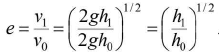• If after n collisions with the ground, the velocity is vn and the height to which it rises be hn, then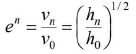During one dimensional collision, when a particle of mass m, moving with velocity U1 collides with another particle of mass m2 at rest, three cases of interest arise:
(i) If m1 = m2, then v1 = 0 and v2 = u1. Under this condition the first particle comes to rest and the second particle moves with the velocity of the first particle before collision. In this case, there occurs maximum transfer of energy.
(ii) If m1 >> m2, then v1 = u1 and v= 2μ1. Under this condition, the velocity of the first particle remains unchanged and the velocity of second particle becomes double that of the first.
(iii) If m1 << m2, then v1 = -u1, and v2 =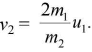v2 is thus approximately zero. Under this condition, the second particle remains at rest while the first particle moves with the same velocity in the opposite direction.
• When m1 = m2 = m but u2 ≠ 0, then v1 = u2 and v2 = u1. The particles mutually exchange their velocities. Exchange of energy is maximum.
• Atomic reactor : Slowing down of neutrons
Hydrogen nuclei and neutrons have almost equal mass. Hence exchange of energy is maximum when they collide. This fact is utilized in atomic reactor for slowing down the fast neutrons.
• Two dimensional elastic collision or oblique collision
(i) The collision between two particles in which the particles do not move along the same straight line before and after the collision is defined as the two dimensional or oblique collision.
Let α1, α2 = angles before collision.
Let β1, β2 = angles after collision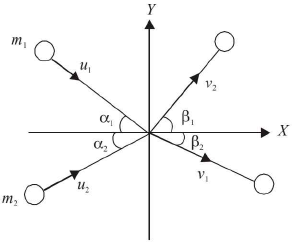(ii) If m1 = m2 and α1 + α2 = 90°
then β1 + β2 = 90°
It means that if two particles of equal mass collide elastically while moving at right angles to each other, then after collision also they move at right angles to each other.
(iii) If a particle A collides elastically with another particle B of equal mass at rest, at a glancing angle, then after the collision the two particles move at right angles to each other i.e. α + β = π/2.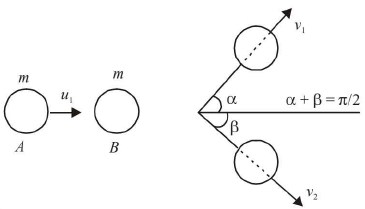• In elastic collision,
- Linear momentum is conserved
- Mechanical energy is conserved.
- For this collision, e = 1.
- The particles do not stick after collision.
- The particles have different velocities after collision.
• In perfectly inelastic collision
- Linear momentum is conserved
- Mechanical energy is not conserved. Infact, the maximum energy loss occurs.
- For this collision, e = 0.
- The particles stick together after collision.
- The particles, after sticking, move with the same velocity after collision.
Offer running on EduRev: Apply code STAYHOME200 to get INR 200 off on our premium plan EduRev Infinity!

,

,

,

,

,

,

,

,

,

,

,

,

,

,

,

,

,

,

,

,

,

,

,

,

;